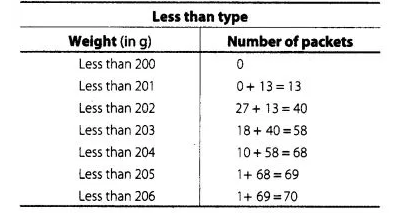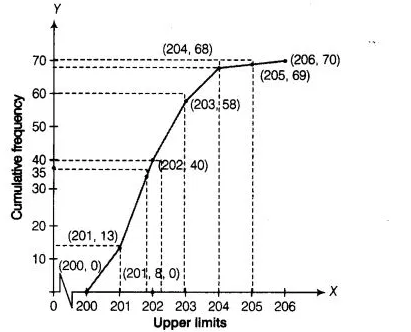# Refer to Q.4 above. Draw the less than type

Question:

Refer to Q.4 above. Draw the less than type ogive for this data and use it to find the median weight.

Solution:

We observe that, the number of packets less than 200 is 0, Similarly, less than 201 include the number of packets from 0-200 as well as the number

of packets from 200-201.

So, the total number of packets less than 201 is 0 + 13 = 13. We say that, the cumulative frequency of the class 200-201 is 13. Similarly, for other

class.To draw the less than type ogive, we plot the points (200, 0), (201, 13), (202, 40) (203, 58), (204, 68), (205, 69) and (206, 70) on the paper and

join by free hand, v Total number of packets (n) = 70Now, $\frac{N}{2}=35$

Firstly, we plot a point (0, 35) on Y-axis and draw a line y = 35 parallel to X-axis. The line cuts the less than ogive curve at a point. We draw a line

on that point which is perpendicular to X-axis. The foot of the line perpendicular to X-axis is the required median.

Median weight = 201.8 g